Java泛型

JAVA技术交流群:737698533

java的泛型并不是新的特性,在JDK1.5时就有的操作,其实我们一直在使用泛型,常见的List,Map中都有它的身影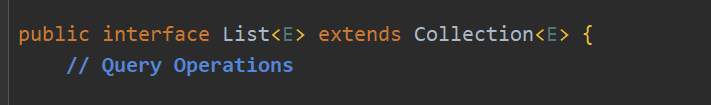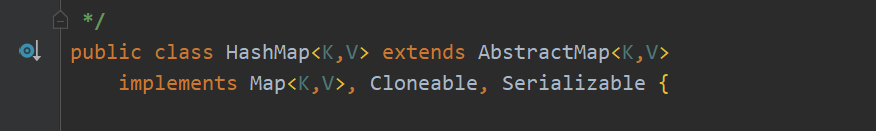public void test1() {
ArrayList arrayList=new ArrayList();

for (Object o : arrayList) {
String name= (String) o;
System.out.println(name);
}
}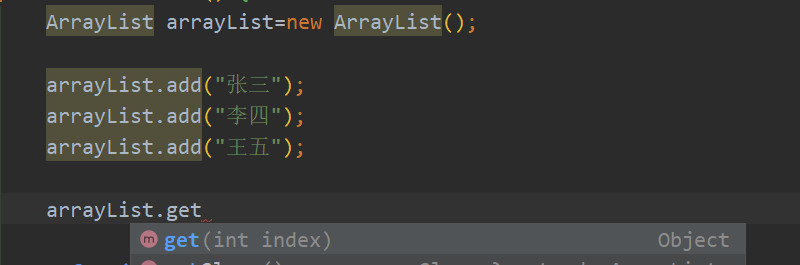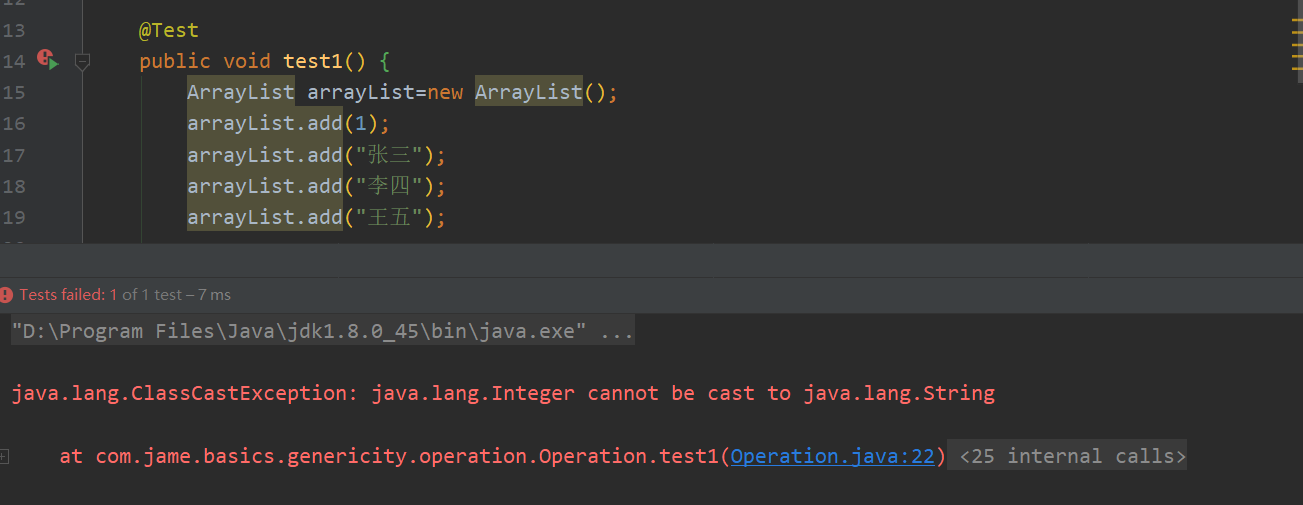public class ArrayList<E> ...{
public E get(int index) {...}
}

ArrayList<String> arrayList=new ArrayList<String>();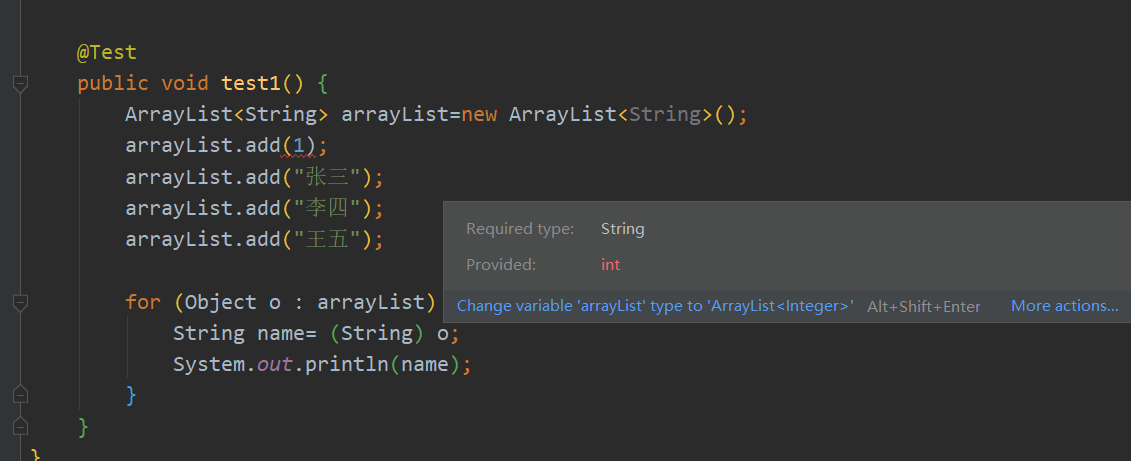idea提示我们所需的类型是一个String类型,而我们提供的是一个int类型,这也说明了泛型具有检查的类型的功能

ArrayList<String> arrayList=new ArrayList<>();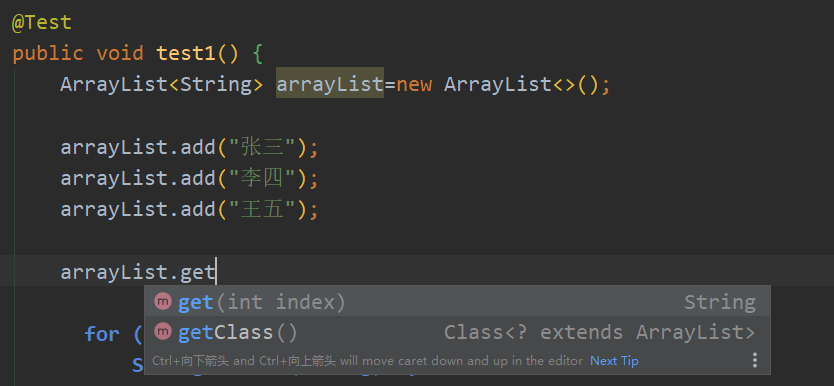public class Hospital<T> {

/**
* 输出动物的信息方法
* @param t
*/
public void print(T t){
System.out.println(t);
}

/**
* 给宠物打疫苗方法
* @return T
*/
public T vaccine(T t){
System.out.println("给宠物打疫苗");
return t;
}
}

public class Cat {

private int age;
private String name;

//省略构造,get,set,toString方法
}

java泛型字母代表意思

E Element (在集合中使用，因为集合中存放的是元素)
T Type（Java 类）
K Key（键）
V Value（值）
N Number（数值类型）
public void test1() {
Hospital<Cat> catHospital = new Hospital<>();
catHospital.print(new Cat(5,"小花"));
}

继承关系

• 如果子类没有保留父类泛型,那么父类泛型默认为Object
• 如果指定父类泛型的类型,那么父类泛型类型为指定的类型
• 如果子类保留了父类类型,那么泛型类型就为子类实例化时设置的类型

//父类
public class Father<A,B> {
}
//子类
public class Son<A,B> extends Father<A,B>{
public void print(A a,B b){
System.out.println("Son-A的类型:"+a.getClass());
System.out.println("Son-B的类型:"+b.getClass());
}
}
//测试
public void test1() {
Son<String, Integer> son = new Son<>();
son.print("",1);
}
//结果
Son-A的类型:class java.lang.String
Son-B的类型:class java.lang.Integer

//父类
public class Father<A,B> {
}
//子类        在这里直接设置了父类泛型类型  ↓
public class Son<A,B> extends Father<Integer,B>{
public void print(A a,B b){
System.out.println("Son-A的类型:"+a.getClass());
System.out.println("Son-B的类型:"+b.getClass());
}
}

//不保留也不指定父类泛型类型            ↓ 去掉这里的 <A,B>
public class Son<A,B> extends Father{
public void print(A a,B b){
System.out.println("Son-A的类型:"+a.getClass());
System.out.println("Son-B的类型:"+b.getClass());
}
}

泛型方法

public <E> E getMax(List<E> es){
if(es==null || es.size()==0)
return null;
E e = es.get(0);
if (e instanceof Integer) {
//获取最大值操作...
}
if(e instanceof String){
//获取最大值操作...
}
return null;
}

//在返回类型前面加上<X> X可以换成任何字母,但是尽量符合规范
//那么在返回类型,传入参数类型,方法体中都可以使用这个定义泛型
public <E> List<E> get(E[] arrays){

}

?通配符

?表示未知类型,

public void test1() {
List<?> list=new ArrayList<>();
//    list.add("小明"); 报错 需要的类型为 ?    而我们传入类型为String
//但是下面这些赋值都没有问题
List<String> stringList = new ArrayList<>();
List<Integer> integerList = new ArrayList<>();
list = stringList;
list = integerList;
}

List<?> 的get方法,获取的类型是Object

//这里使用迭代器来进行遍历
public void print(List<?> list){
ListIterator<?> listIterator = list.listIterator();
while (listIterator.hasNext()){
Object next = listIterator.next();
System.out.println(next);
}
}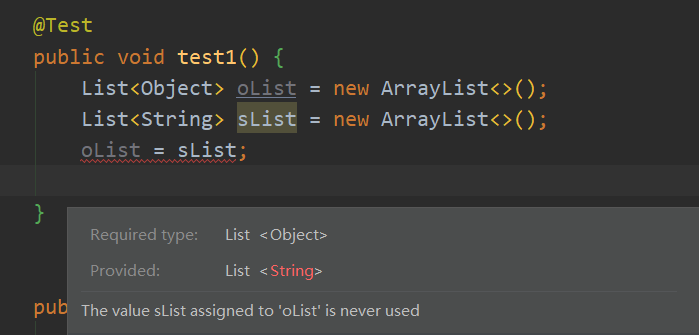String为Object的子类,为什么不能赋值给Object的集合呢?

通配符限制条件

<? extends xxx><? super xxx>

Animal动物类为父类->Cat猫类继承Animal类-> XiaoHuaCat小花猫类继承Cat类

@Test
public void test1() {
//设置通配符限制条件
List<? extends Cat> list = new ArrayList<>();
List<Cat> cats = new ArrayList<>();
List<XiaoHuaCat> xiaoHuaCats = new ArrayList<>();
List<Animal> animals = new ArrayList<>();

list = cats;
list = xiaoHuaCats;
//这条赋值语句报错,因为不是继承Cat或Cat类型
list = animals;
}

@Test
public void test1() {
List<? super Cat> list = new ArrayList<>();
List<Cat> cats = new ArrayList<>();
List<Animal> animals = new ArrayList<>();
List<XiaoHuaCat> xiaoHuaCats = new ArrayList<>();

list = cats;
list = animals;
//这条语句会报错,因为最小的类型就是Cat类了,而XiaoHuaCat是继承于Cat的
list = xiaoHuaCats;
}

posted @ 2020-12-31 23:33  Jame!  阅读(115)  评论(0编辑  收藏  举报